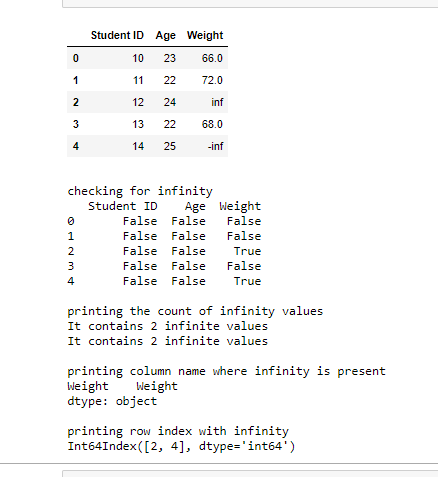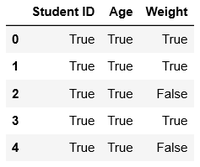Open In App

# Check if dataframe contains infinity in Python – Pandas

Prerequisites: Pandas

There are various cases where a data frame can contain infinity as value. This article discusses how we can keep track of infinities in our data frame.

### Approach

• Import module
• Create a data frame, for this article, it is done using a dictionary. For including infinity in the data, import NumPy module, and use np.inf for positive infinity and -np.inf for negative infinity.
• Use appropriate methods from the ones mentioned below as per your requirement.

Method 1: Use DataFrame.isinf() function to check whether the dataframe contains infinity or not. It returns boolean value. If it contains any infinity, it will return True. Else, it will return False.

Syntax:

`isinf(array [, out])`

Using this method itself, we can derive a lot more information regarding the presence of infinity in our dataframe:

• Checking for infinity as values
• Counting the number of infinity values
• Retrieve column name with infinity as value(s)
• Retrieve row index/indices with infinity as value(s)

Example:

## Python3

 `# Import required libraries`` ` `import` `pandas as pd``import` `numpy as np`` ` `# Create dataframe using dictionary``data ``=` `{``'Student ID'``: [``10``, ``11``, ``12``, ``13``, ``14``], ``        ``'Age'``: [``23``, ``22``, ``24``, ``22``, ``25``],``        ``'Weight'``: [``66``, ``72``, np.inf, ``68``, ``-``np.inf]}`` ` `df ``=` `pd.DataFrame(data)`` ` `display(df)`` ` `# checking for infinity``print``()``print``(``"checking for infinity"``)`` ` `ds ``=` `df.isin([np.inf, ``-``np.inf])``print``(ds)`` ` `# printing the count of infinity values``print``()``print``(``"printing the count of infinity values"``)`` ` `count ``=` `np.isinf(df).values.``sum``()``print``(``"It contains "` `+` `str``(count) ``+` `" infinite values"``)`` ` `# counting infinity in a particular column name``c ``=` `np.isinf(df[``'Weight'``]).values.``sum``()``print``(``"It contains "` `+` `str``(c) ``+` `" infinite values"``)`` ` `# printing column name where infinity is present``print``()``print``(``"printing column name where infinity is present"``)``col_name ``=` `df.columns.to_series()[np.isinf(df).``any``()]``print``(col_name)`` ` `# printing row index with infinity``print``()``print``(``"printing row index with infinity "``)`` ` `r ``=` `df.index[np.isinf(df).``any``(``1``)]``print``(r)`

Output:Method 2: Use np.isfinite(dataframe_name) to check the presence of infinite value(s). It returns boolean value. It will return False for infinite values and it will return True for finite values.

Syntax:

`isfinite(array [, out])`

Example:

## Python3

 `# Import required libraries`` ` `import` `pandas as pd``import` `numpy as np`` ` `# Create dataframe using dictionary``data ``=` `{``'Student ID'``: [``10``, ``11``, ``12``, ``13``, ``14``], ``'Age'``: [``    ``23``, ``22``, ``24``, ``22``, ``25``], ``'Weight'``: [``66``, ``72``, np.inf, ``68``, ``-``np.inf]}`` ` `df ``=` `pd.DataFrame(data)`` ` `d ``=` `np.isfinite(df)`` ` `display(d)`

Output: